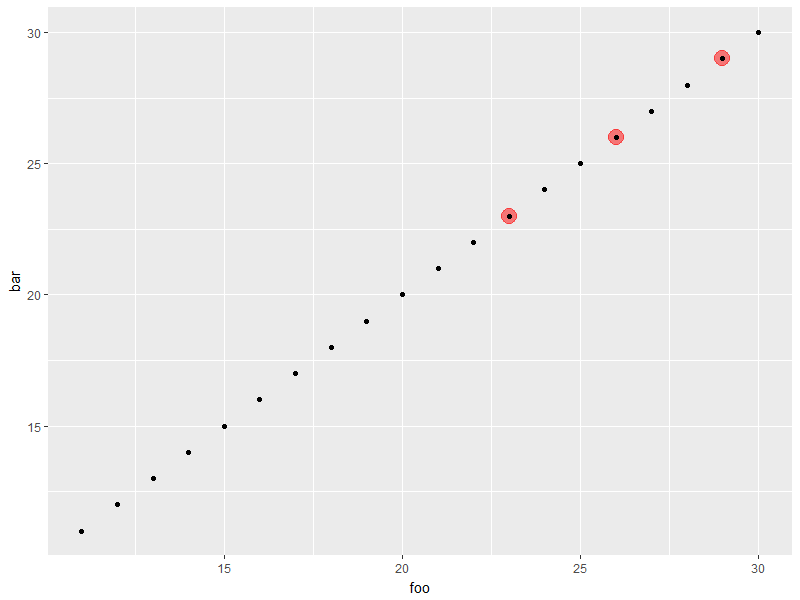# An Example Usage of ggplot_add()

gghighlight
ggplot2
R package
Author

Hiroaki Yutani

Published

November 7, 2017

A generic function `ggplot_add()` was added to ggplot2 by this PR:

Allow addition of custom objects by thomasp85 · Pull Request #2309 · tidyverse/ggplot2

I think creating a custom `Geom` or `Stat` and its constructor (`geom_*()` or `stat_*()`) is enough for the most of the extension packages of ggplot2, but some people, including me, need this.

## Why are there no `geom_highlight()`?

Here is an example code of my package gghighlight:

``gghighlight_point(d, aes(foo, bar), predicate = bar > 20 & baz == "A")``

You may wonder why this can’t be written like this:

``````ggplot(d, aes(foo, bar)) +
geom_highlight_point(bar > 20 & baz == "A")``````

Let me explain a bit.

## `geom_*()`/`stat_*()` doesn’t know about the other layers

`geom_highlight_point(bar > 20 & baz == "A")` is passed `bar > 20 & baz == "A"` without the data `d`, with which the expression should be evaluated. It needs `d` specified in `ggplot(...)`.

But, considering the structure of the above code, `geom_highlight_point(...)` cannot access to the result of `ggplot(...)` in any usual way:

```+`(ggplot(...), geom_highlight_point(...))``

If ggplot2 were designed pipe-friendly, this

```%>%`(ggplot(...), geom_highlight_point(...))``

would be evaluated as this, which means `geom_highlight_point(...)` could take `d` from `ggplot(...)`

``geom_highlight_point(ggplot(...), ...)``

Anyway, let’s give up here. All I have to do is set this expression as an attribute of a custom `Geom` and pray that it will be evaluated with the proper data in the phase of building a plot.

## `Geom*`/`Stat*` doesn’t know about the original data

Take a look at the simplest example in the vignette “Extending ggplot2”:

``````StatChull <- ggproto("StatChull", Stat,
compute_group = function(data, scales) {
data[chull(data\$x, data\$y), , drop = FALSE]
},

required_aes = c("x", "y")
)``````

You may notice that `compute_group()` expects `data` has the fixed column `x` and `y`. Actually, `data` is not the original data but the one the mapping is already applied. So, there is no column `bar` and `baz` anymore; `bar` is renamed to `y` and `baz` is dropped. Oh no, I cannot evaluate `bar > 20 & baz == "A"` here, too…

## `ggplot_add()`

Let’s remember this one:

```+`(ggplot(...), geom_highlight_point(...))``

Only `+` (or `+.gg`) can access to both `ggplot(...)` and `geom_highlight_point(...)`. This means that, inside `+.gg`, `bar > 20 & baz == "A"` can be evaluated.

So…, should I implement my custom `+.gg` by myself? No, because I will be able to use `ggplot_add()`!

`ggplot_add()` is called in `+.gg()` via `add_ggplot()` (very confusing..) and is passed both the original plot and the new object. The current implementation is this:

``````"+.gg" <- function(e1, e2) {
# Get the name of what was passed in as e2, and pass along so that it
# can be displayed in error messages
e2name <- deparse(substitute(e2))

if      (is.theme(e1))  add_theme(e1, e2, e2name)
else if (is.ggplot(e1)) add_ggplot(e1, e2, e2name)
else if (is.ggproto(e1)) {
stop("Cannot add ggproto objects together.",
" Did you forget to add this object to a ggplot object?",
call. = FALSE)
}
}``````

(https://github.com/tidyverse/ggplot2/blob/7d0549a03e5ea08c27c768e88d5717f18cb4a5ce/R/plot-construction.r#L40-L52)

``````add_ggplot <- function(p, object, objectname) {
if (is.null(object)) return(p)

p <- plot_clone(p)
p <- ggplot_add(object, p, objectname)
set_last_plot(p)
p
}``````

(https://github.com/tidyverse/ggplot2/blob/7d0549a03e5ea08c27c768e88d5717f18cb4a5ce/R/plot-construction.r#L59-L66)

By using this, I can implement the proof-of-concept version of `geom_highlight_point()` as bellow:

``````geom_highlight_point <- function(expr) {
structure(list(expr = rlang::enquo(expr)), class = "highlight")
}

ggplot_add.highlight <- function(object, plot, object_name) {
new_data <- dplyr::filter(plot\$data, !! object\$expr)
new_layer <- geom_point(data = new_data,
mapping = plot\$mapping,
colour = alpha("red", 0.5),
size = 5)
plot\$layers <- append(plot\$layers, new_layer)
plot
}``````
``````library(ggplot2)

d <- data.frame(foo = 11:30, bar = 11:30, baz = rep(c("A", "B", "C"), length.out = 20),
stringsAsFactors = FALSE)

ggplot(d, aes(foo, bar)) +
geom_highlight_point(bar > 20 & baz == "A") +
geom_point()  # for comparison``````I’m not sure if this is the intended usage of `ggplot_add()`, but this seems very nice. Looking forward to the next release of ggplot2!